# Selina Solutions Concise Maths Class 10 Chapter 20 Cylinder, Cone and Sphere (Surface Area and Volume) Exercise 20(F)

Miscellaneous problems of this chapter are well structured under this exercise. Students wishing to learn the right methods of solving problems of this book can have a quick reference at the Selina Solutions for Class 10 Maths. All the solutions are prepared by subject experts at BYJU’S and are on par with the latest ICSE marking schemes. Also, the solutions to the Concise Selina Solutions for Class 10 Maths Chapter 20 Cylinder, Cone and Sphere (Surface Area and Volume) Exercise 20(F) PDF can be accessed from the link given below.

## Selina Solutions Concise Maths Class 10 Chapter 20 Cylinder, Cone and Sphere (Surface Area and Volume) Exercise 20(F) Download PDF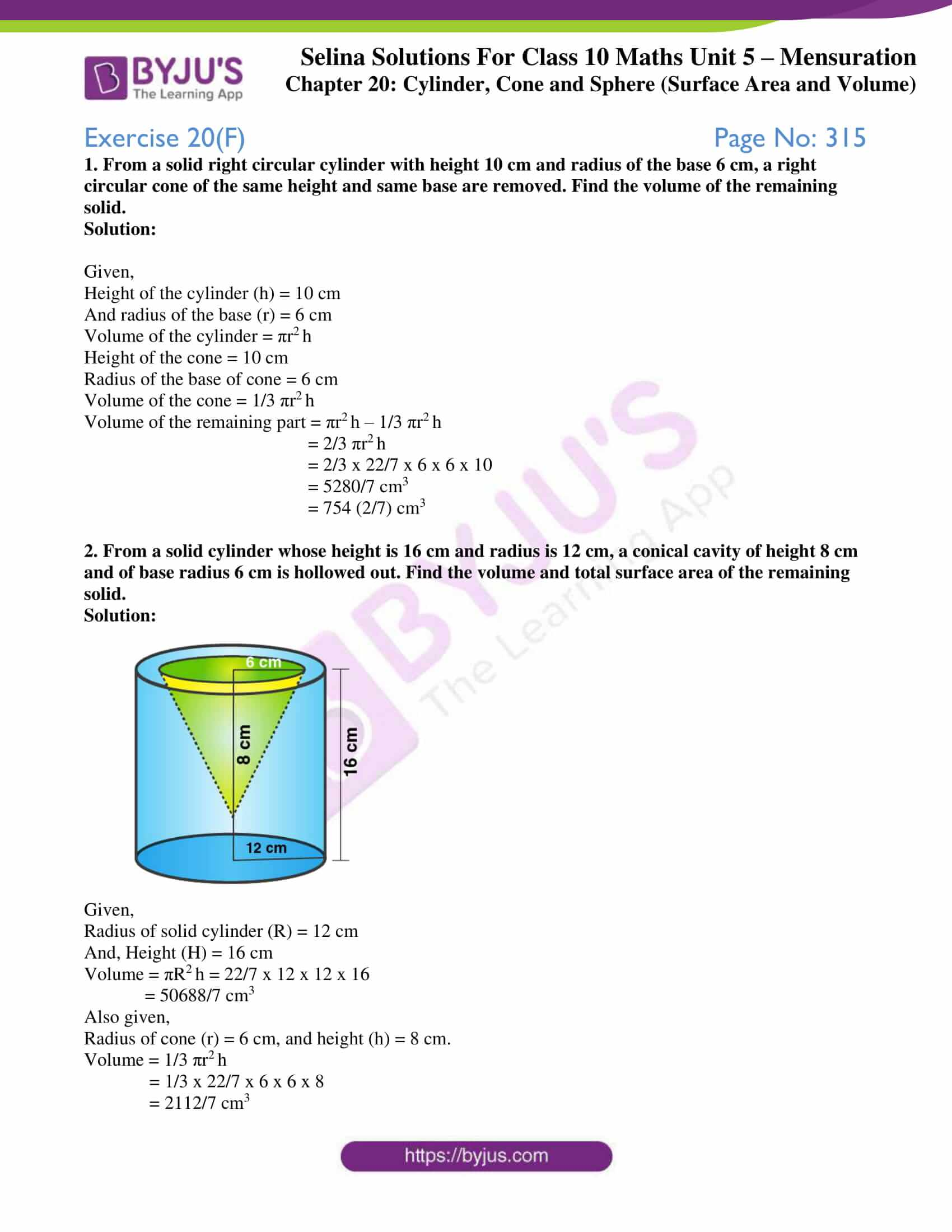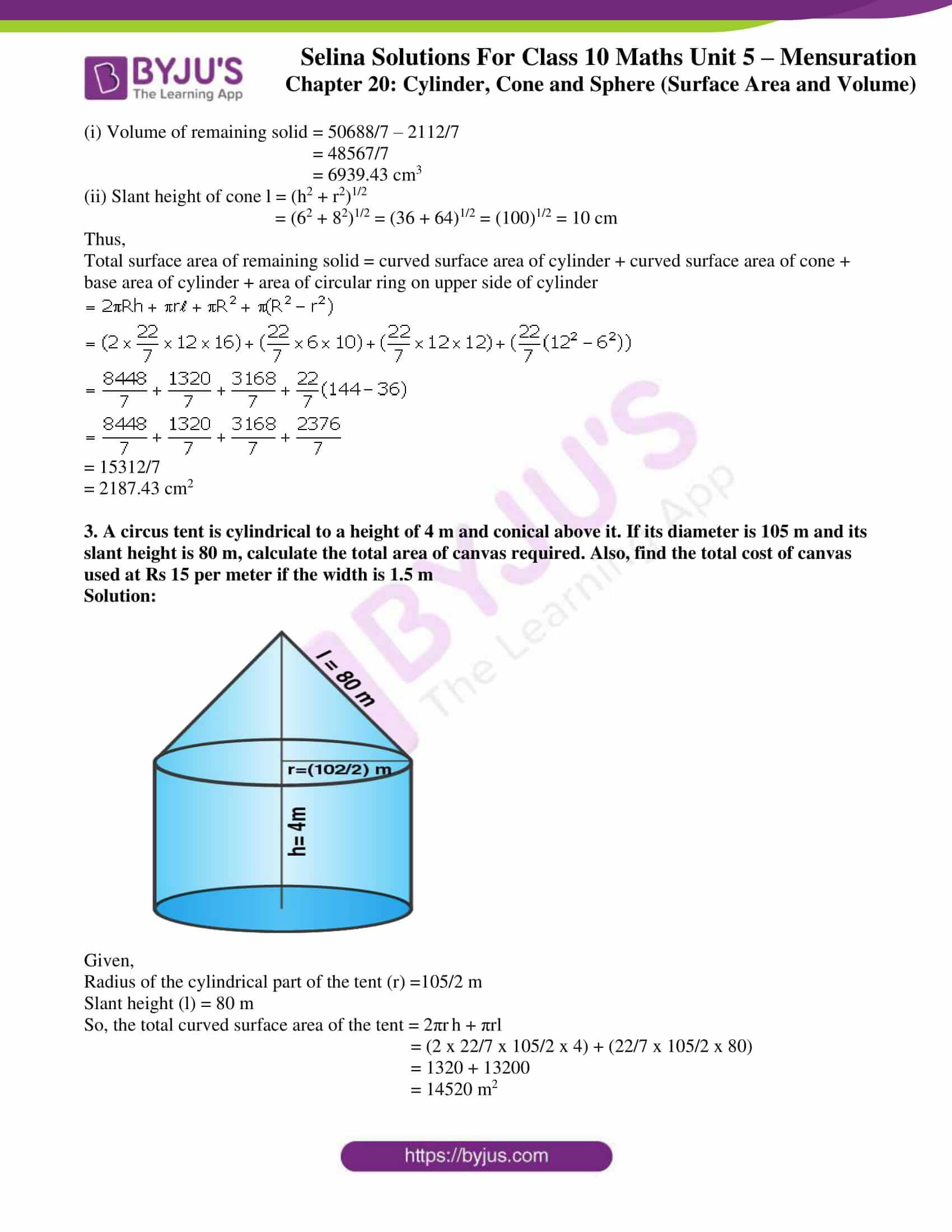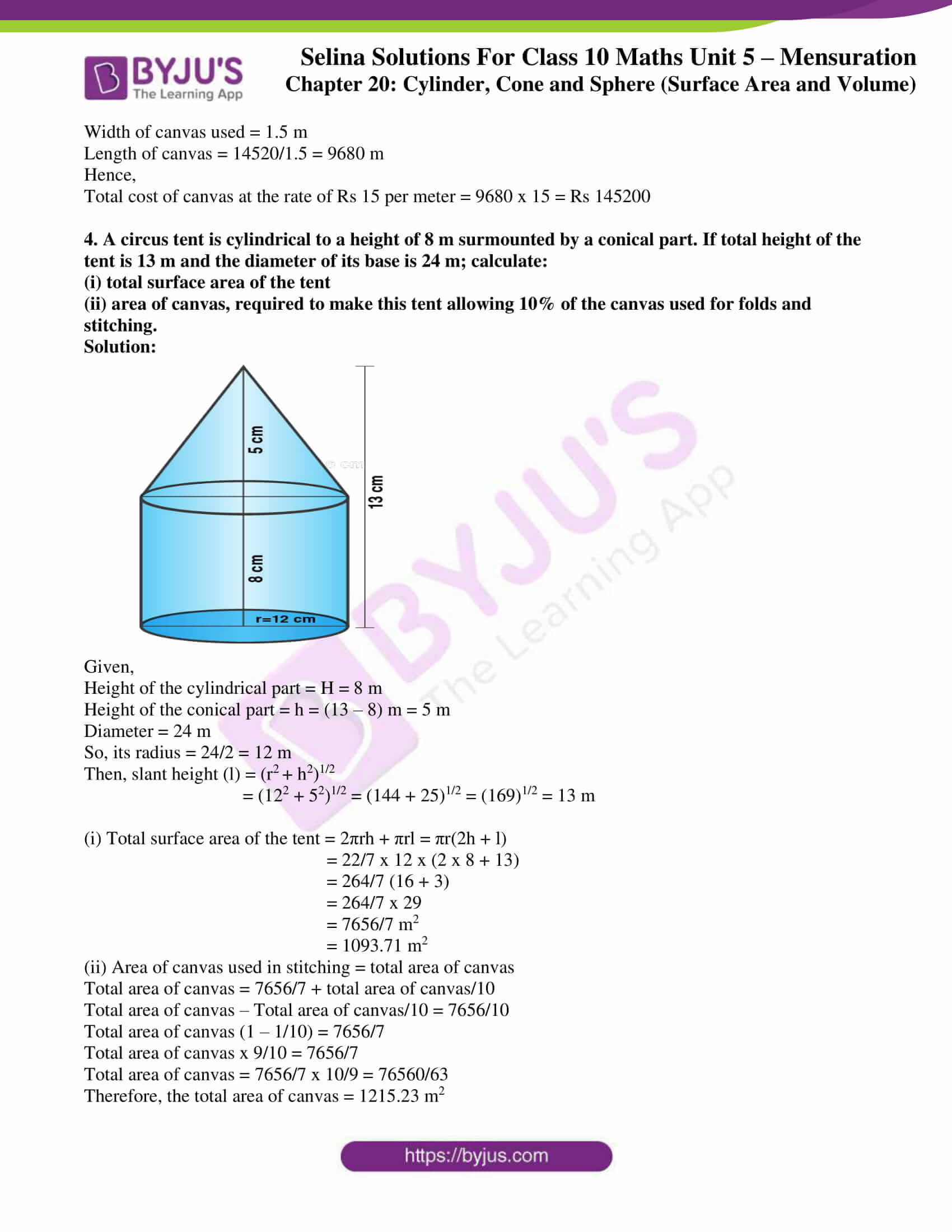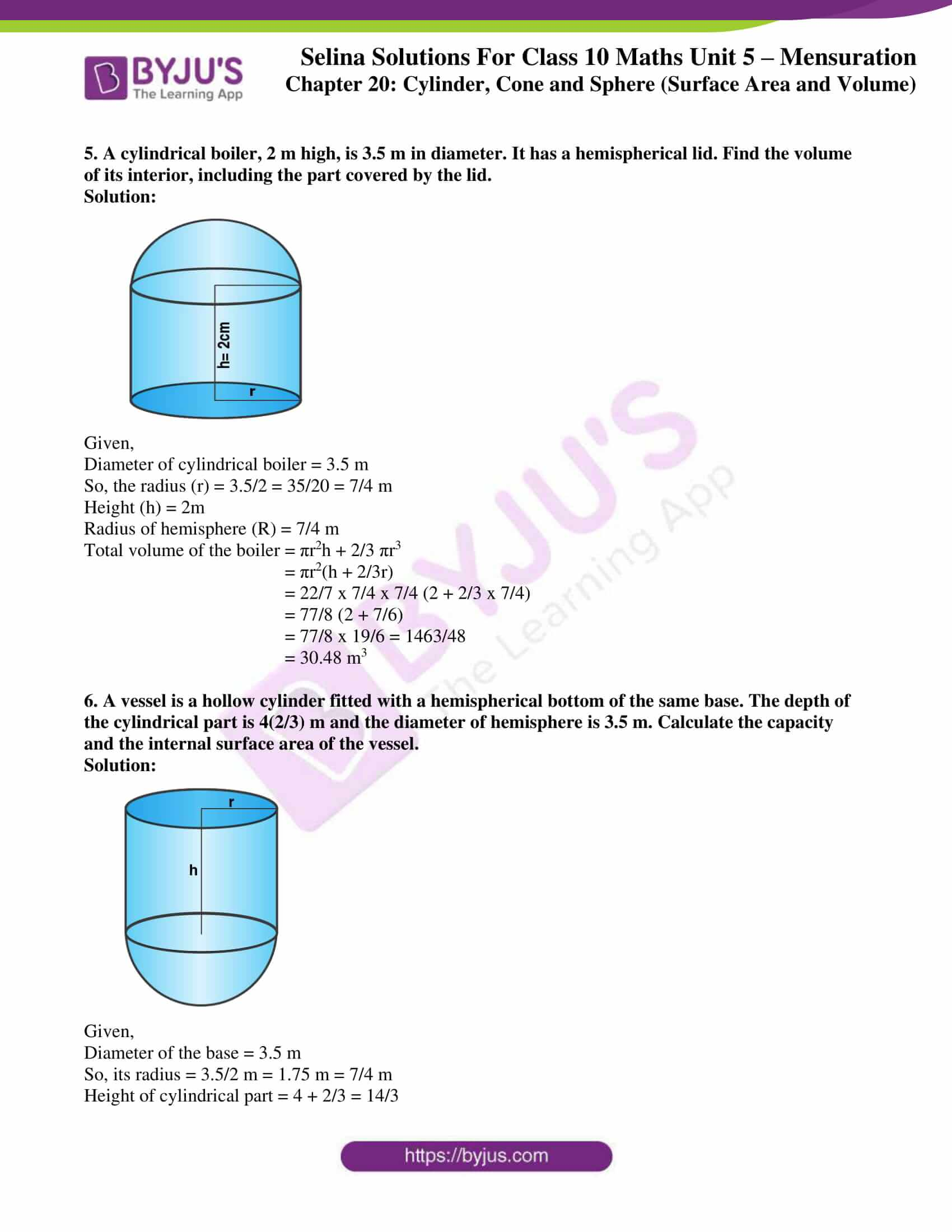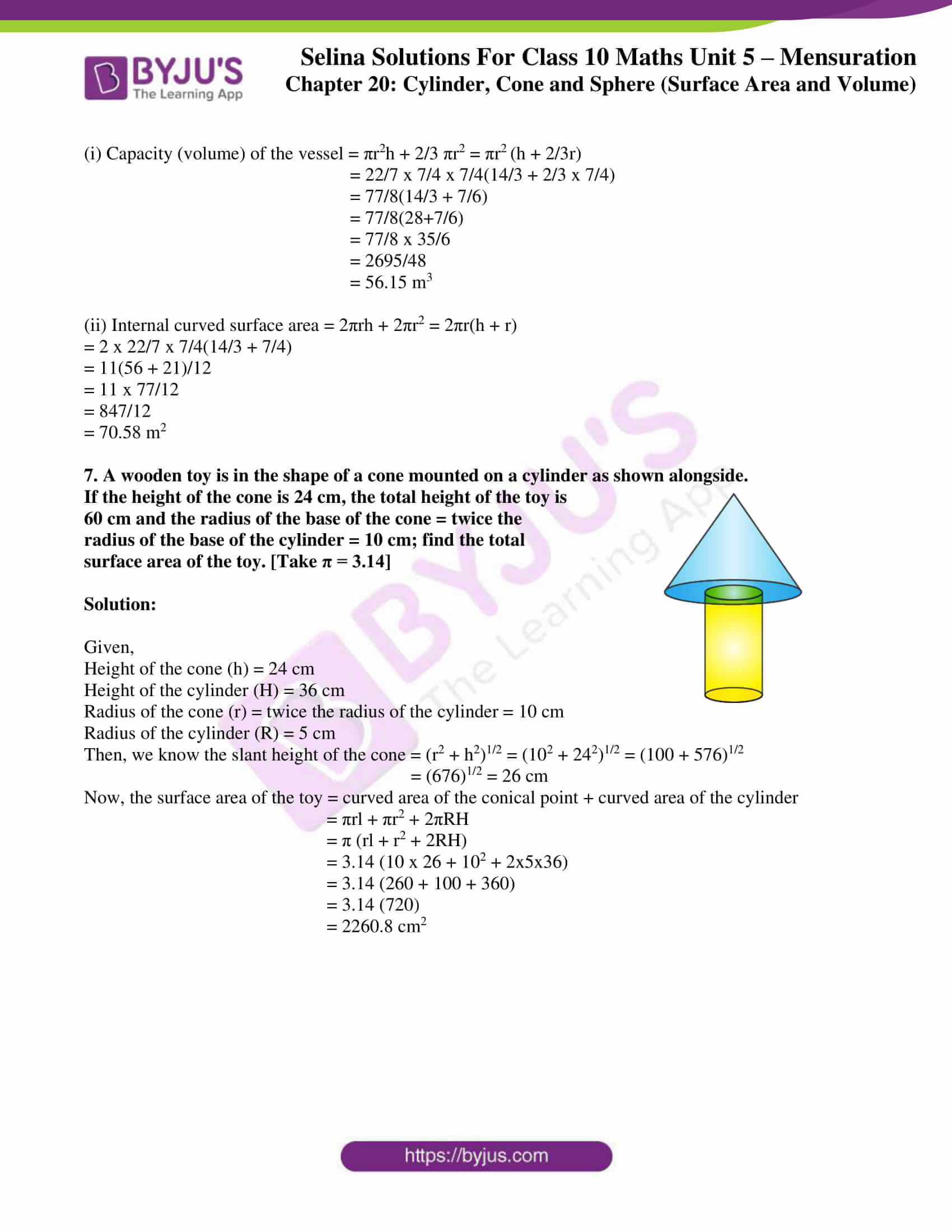### Access Selina Solutions Concise Maths Class 10 Chapter 20 Cylinder, Cone and Sphere (Surface Area and Volume) Exercise 20(F)

1. From a solid right circular cylinder with height 10 cm and radius of the base 6 cm, a right circular cone of the same height and same base are removed. Find the volume of the remaining solid.

Solution:

Given,

Height of the cylinder (h) = 10 cm

And radius of the base (r) = 6 cm

Volume of the cylinder = πr2 h

Height of the cone = 10 cm

Radius of the base of cone = 6 cm

Volume of the cone = 1/3 πr2 h

Volume of the remaining part = πr2 h – 1/3 πr2 h

= 2/3 πr2 h

= 2/3 x 22/7 x 6 x 6 x 10

= 5280/7 cm3

= 754 (2/7) cm3

2. From a solid cylinder whose height is 16 cm and radius is 12 cm, a conical cavity of height 8 cm and of base radius 6 cm is hollowed out. Find the volume and total surface area of the remaining solid.

Solution: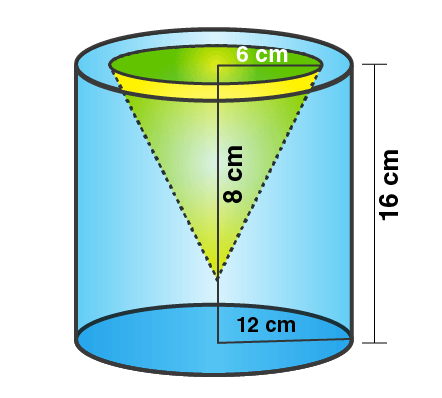Given,

Radius of solid cylinder (R) = 12 cm

And, Height (H) = 16 cm

Volume = πR2 h = 22/7 x 12 x 12 x 16

= 50688/7 cm3

Also given,

Radius of cone (r) = 6 cm, and height (h) = 8 cm.

Volume = 1/3 πr2 h

= 1/3 x 22/7 x 6 x 6 x 8

= 2112/7 cm3

(i) Volume of remaining solid = 50688/7 – 2112/7

= 48567/7

= 6939.43 cm3

(ii) Slant height of cone l = (h2 + r2)1/2

= (62 + 82)1/2 = (36 + 64)1/2 = (100)1/2 = 10 cm

Thus,

Total surface area of remaining solid = curved surface area of cylinder + curved surface area of cone + base area of cylinder + area of circular ring on upper side of cylinder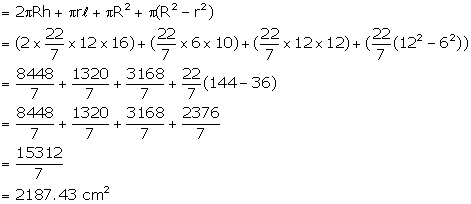= 15312/7

= 2187.43 cm2

3. A circus tent is cylindrical to a height of 4 m and conical above it. If its diameter is 105 m and its slant height is 80 m, calculate the total area of canvas required. Also, find the total cost of canvas used at Rs 15 per meter if the width is 1.5 m

Solution: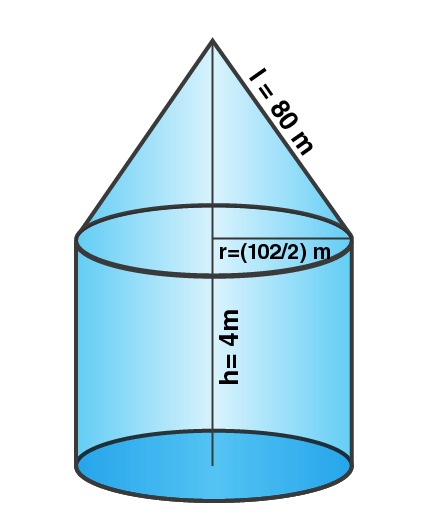Given,

Radius of the cylindrical part of the tent (r) =105/2 m

Slant height (l) = 80 m

So, the total curved surface area of the tent = 2πr h + πrl

= (2 x 22/7 x 105/2 x 4) + (22/7 x 105/2 x 80)

= 1320 + 13200

= 14520 m2

Width of canvas used = 1.5 m

Length of canvas = 14520/1.5 = 9680 m

Hence,

Total cost of canvas at the rate of Rs 15 per meter = 9680 x 15 = Rs 145200

4. A circus tent is cylindrical to a height of 8 m surmounted by a conical part. If total height of the tent is 13 m and the diameter of its base is 24 m; calculate:

(i) total surface area of the tent

(ii) area of canvas, required to make this tent allowing 10% of the canvas used for folds and stitching.

Solution: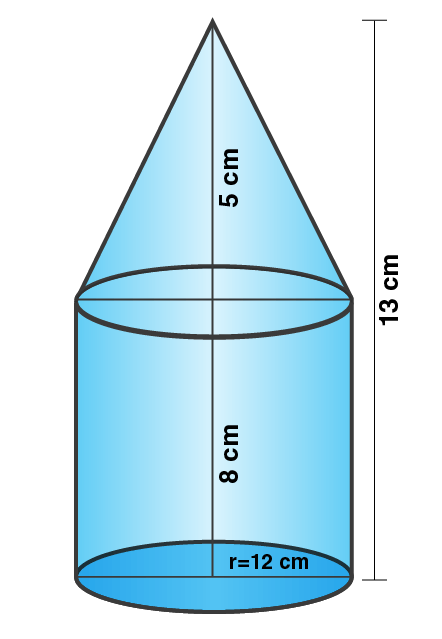Given,

Height of the cylindrical part = H = 8 m

Height of the conical part = h = (13 – 8) m = 5 m

Diameter = 24 m

So, its radius = 24/2 = 12 m

Then, slant height (l) = (r2 + h2)1/2

= (122 + 52)1/2 = (144 + 25)1/2 = (169)1/2 = 13 m

(i) Total surface area of the tent = 2πrh + πrl = πr(2h + l)

= 22/7 x 12 x (2 x 8 + 13)

= 264/7 (16 + 3)

= 264/7 x 29

= 7656/7 m2

= 1093.71 m2

(ii) Area of canvas used in stitching = total area of canvas

Total area of canvas = 7656/7 + total area of canvas/10

Total area of canvas – Total area of canvas/10 = 7656/10

Total area of canvas (1 – 1/10) = 7656/7

Total area of canvas x 9/10 = 7656/7

Total area of canvas = 7656/7 x 10/9 = 76560/63

Therefore, the total area of canvas = 1215.23 m2

5. A cylindrical boiler, 2 m high, is 3.5 m in diameter. It has a hemispherical lid. Find the volume of its interior, including the part covered by the lid.

Solution: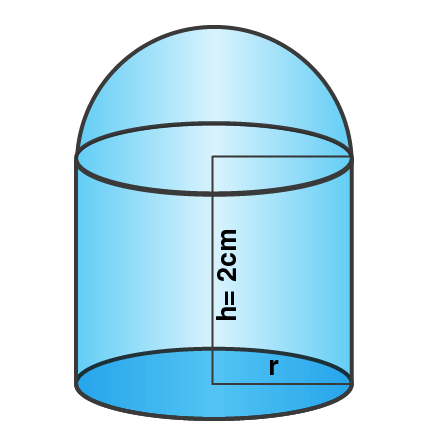Given,

Diameter of cylindrical boiler = 3.5 m

So, the radius (r) = 3.5/2 = 35/20 = 7/4 m

Height (h) = 2m

Radius of hemisphere (R) = 7/4 m

Total volume of the boiler = πr2h + 2/3 πr3

= πr2(h + 2/3r)

= 22/7 x 7/4 x 7/4 (2 + 2/3 x 7/4)

= 77/8 (2 + 7/6)

= 77/8 x 19/6 = 1463/48

= 30.48 m3

6. A vessel is a hollow cylinder fitted with a hemispherical bottom of the same base. The depth of the cylindrical part is 4(2/3) m and the diameter of hemisphere is 3.5 m. Calculate the capacity and the internal surface area of the vessel.

Solution: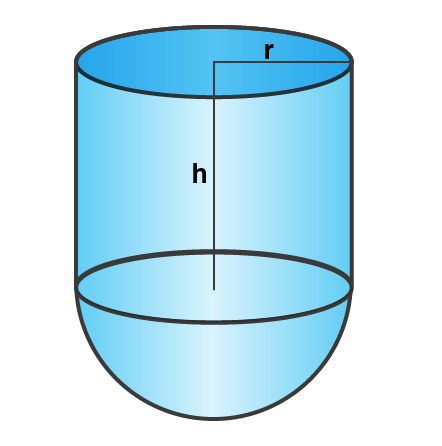Given,

Diameter of the base = 3.5 m

So, its radius = 3.5/2 m = 1.75 m = 7/4 m

Height of cylindrical part = 4 + 2/3 = 14/3

(i) Capacity (volume) of the vessel = πr2h + 2/3 πr2 = πr2 (h + 2/3r)

= 22/7 x 7/4 x 7/4(14/3 + 2/3 x 7/4)

= 77/8(14/3 + 7/6)

= 77/8(28+7/6)

= 77/8 x 35/6

= 2695/48

= 56.15 m3

(ii) Internal curved surface area = 2πrh + 2πr2 = 2πr(h + r)

= 2 x 22/7 x 7/4(14/3 + 7/4)

= 11(56 + 21)/12

= 11 x 77/12

= 847/12

= 70.58 m2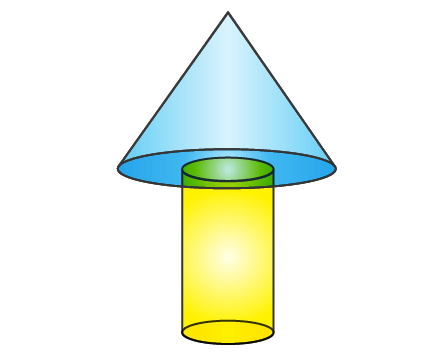7. A wooden toy is in the shape of a cone mounted on a cylinder as shown alongside.

If the height of the cone is 24 cm, the total height of the toy is 60 cm and the radius of the base of the cone = twice the radius of the base of the cylinder = 10 cm; find the total surface area of the toy. [Take π = 3.14]

Solution:

Given,

Height of the cone (h) = 24 cm

Height of the cylinder (H) = 36 cm

Radius of the cone (r) = twice the radius of the cylinder = 10 cm

Radius of the cylinder (R) = 5 cm

Then, we know the slant height of the cone = (r2 + h2)1/2 = (102 + 242)1/2 = (100 + 576)1/2

= (676)1/2 = 26 cm

Now, the surface area of the toy = curved area of the conical point + curved area of the cylinder

= πrl + πr2 + 2πRH

= π (rl + r2 + 2RH)

= 3.14 (10 x 26 + 102 + 2x5x36)

= 3.14 (260 + 100 + 360)

= 3.14 (720)

= 2260.8 cm2